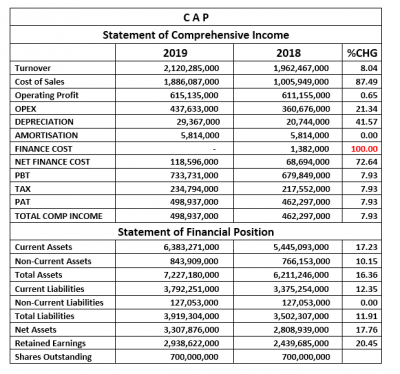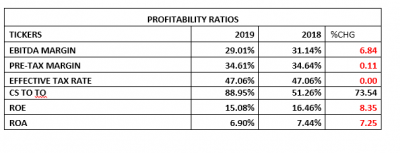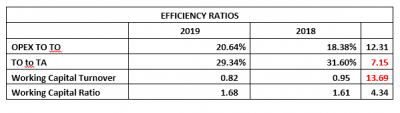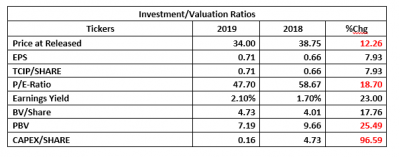Company Analysis

# CAP Plc 2019Q1: Time To Expand For More Attractive Valuation

Coy: CAP Plc
Rating: Hold
Current Market Price: N31.10
Year High: N37.40
Year Low: N31.10
Fair Value: N33.26
Equity Analyst: Tunde Segun Jeariogbe

Key Investment Ratios
• This report observed the operations of Chemical & Allied Product Plc for the first three months of 2019, compared it with the similar quarter of 2018 to establish growth pattern. While attempting to rightly price each unit of the company, the 2018 full year financial statistics were analyzed.
• As at the end of the review period, the company did not have any interest yielding liability and as such posted a nil Finance Cost, compared to the N1.38bn posted in the similar period of 2018 and N14.61bn at the end of 2018 full year.
• Please note that the only item carried on the long term liabilities column of the result is Deferred Tax Liabilities amounting to N127.05 million, same as that of the corresponding quarter of 2018.
• As good as this position is, it could also be viewed as an equilibrium state for the business, which means that building earnings above the previous year’s might be stiff since the company is now using zero debt to create more wealth.
• Based on our conservative valuation approach, we have rated the shares a ‘Hold,’ with a conclusion that the company will need to aggressively expand its business space with the aim of creating wealth for its shareholders.Company figures
• The turnover figure improved by a marginal 8.04% over that of the comparable period of 2018, moving from N1.96 billion to N2.12 billion.
• Cost of Sales increased at a higher pace as the difference between the current and preceding quarter of 2018 is estimated at 87.49%.
• With higher operating expenses, the Operating Profit rose by a marginal 0.65% above that of 2018 first quarter to N615.13 million, as against the N611.15 million achieved in 2018 first quarter.
• As noted previously, Operating Expenses stood at N437.63 million, same as 21.34% above N360.67 million posted in 2018Q1.
• As mentioned above, the company posted a zero Finance Cost at the end of the quarter, this is against the N1.38 million of similar quarter
• Meanwhile, Net Finance Cost was positive N118.59 million, which is 72.64% above 2018 first quarter estimate.
• Both Profits before and After-Tax rose above the corresponding period by 7.93% each. Current Profit before tax is estimated at N733.73 million while Profit for the year stood at N498.93 million.Financial Strength/Solvency Ratios
• Debt Ratio is currently estimated at 0.54x, down from the 0.56x in the 2018 first quarter financials, implying that Total Liabilities is same as 54% of Total Assets. Note also that the quality of this ratio is based on its zero long-term/interest yielding liabilities.
• Total Debt to Equity was equally estimated at 1.18x as against 1.25x estimated in the comparable quarter of the prior year.
• It was also estimated that Total Equity is same as 46% of the company’s Total Assets, which is 1.21% improvement over the 45% estimated last quarter.

• The turnover figure improved by a marginal 8.04% over that of the comparable period of 2018, moving from N1.96 billion to N2.12 billion.
• Cost of Sales increased at a higher pace as the difference between the current and preceding quarter of 2018 is estimated at 87.49%.
• With higher operating expenses, the Operating Profit rose by a marginal 0.65% above that of 2018 first quarter to N615.13 million, as against the N611.15 million achieved in 2018 first quarter.
• As noted previously, Operating Expenses stood at N437.63 million, same as 21.34% above N360.67 million posted in 2018Q1.
• As mentioned above, the company posted a zero Finance Cost at the end of the quarter, this is against the N1.38 million of similar quarter
• Meanwhile, Net Finance Cost was positive N118.59 million, which is 72.64% above 2018 first quarter estimate.
• Both Profits before and After-Tax rose above the corresponding period by 7.93% each. Current Profit before tax is estimated at N733.73 million while Profit for the year stood at N498.93 million.

Financial Strength/Solvency Ratios
• Debt Ratio is currently estimated at 0.54x, down from the 0.56x in the 2018 first quarter financials, implying that Total Liabilities is same as 54% of Total Assets. Note also that the quality of this ratio is based on its zero long-term/interest yielding liabilities
• Total Debt to Equity was equally estimated at 1.18x as against 1.25x estimated in the comparable quarter of prior year.
• It was also estimated that Total Equity is same as 46% of the company’s Total Assets, which is 1.21% improvement over the 45% estimated last quarter.Profitability Ratios
• EBITDA margin estimated for the period stood at 29.01%, 6.84% below the 31.14% estimated from the corresponding quarter’s numbers.
• Pre-Tax Margin dropped marginally by 0.11% to 34.16%, as against the 34.64% estimated at the end of 2018 first quarter.
• Effective Tax Rate was stable within the two periods compared in this report.
• The company seems to have operated with higher cost through the quarter as Cost of Sales represented 88.95% of Turnover, up by 73.54% over the 51.26% CoS to Turnover of 2018 first quarter.
• Return on Equity stood at 15.08% against 16.46% of 2018.
• Similarly, Return on Assets dropped by 7.25% to stand at 6.90% as against 7.44% of 2018 first quarter.Efficiency Ratios
• Operating Expenses to Turnover value is same as 20.64%, this is 12.31% growth over the 18.38% estimated from 2018 first quarter financials
• Turnover to Total Assets Ratios stood at 29.34%, as against the previous 31.60%, representing 7.15% drop in efficiency over the comparable period of 2018.
• Working Capital Ratio which indicates a company’s effectiveness in the use of its working capital was estimated at 0.82x a 13.69% drop from the 0.95x estimated in the similar period of 2018.
• To further test the company’s efficiency, we used the Working Capital Ratio, which confirmed that Current Assets is higher than current liabilities. We also established improvement in this efficiency ratio from the previous 1.61x to 1.68x.Investment/Valuation Ratios
• At the end of the first three months of 2019, the management achieved earnings per share improvement to the tune of 7.93%, from the previously estimated N0.66 to N0.71.
• P/E-Ratio slipped by 18.70%, moving from 47.70x to 58.67x. We must understand that the high P/E-Ratio is an indication of positive investors’ sentiments.
• The said Earnings per Share represents 2.10% yield of the current market price of CAP Plc shares on the floor of the Nigerian Stock Exchange as at the time the result was presented.
• Further confirming the positive sentiments is the low Book Value of N4.73, as against the market price of N34.00.
• Due to the very low Book Value of CAP Plc shares as against the market value, the Price to Book Value is quite high at 7.19x as against 9.66x estimated from the corresponding
• Although Capex/Share estimate dropped by 96.59% from the comparable period estimate, the ratio is still very positive, confirming its strength to fund its dividend payments.Valuation
Our blend of valuation models considered the consistent dividend payment of CAP Plc and stability. We also put into consideration the seeming stability in the performance indices over the period. Thus we valued each unit of CAP Plc shares at N33.26, slightly below the current market valuation of the shares as at the time the latest financials were made available to the investing public. We have therefore rated it a Hold.

Check Also
Close